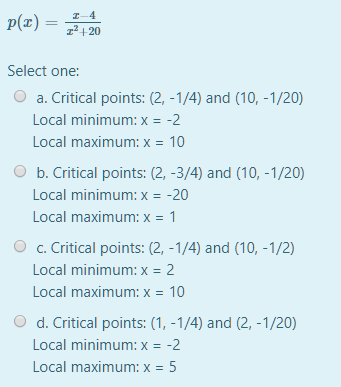which one

reopened

dp/dx=(x²+20)-2x(x-4))/(x²+20)²=0 at an extremum, dp/dx=1/(x²+20)-2x(x-4)/(x²+20)².

That is, x²+20-2x²+8x=0, -x²+8x+20=0=-(x-10)(x+2). The critical points are at x=10 and -2.

d²p/dx²=-2x/(x²+20)²-[(x²+20)²(4x-8)-4x(2x²-8x)(x²+20)]/(x²+20)⁴.

When x=10 this is -20/120²-[120²(32)-40(120)²]/120⁴=-20/120²+8/120²<0 (maximum), and p(10)=6/120=1/20.

When x=-2 it’s 4/24²-[24²(-16)+8(24²)]/24⁴>0 (minimum), and p(-2)=-6/24=-¼.

Max at (10,1/20); min at (-2,-¼). If the critical points are the maximum and minimum then all the answer options are incorrect, the closest being answer a. But the points in this answer option are not even on the curve!

by Top Rated User (1.1m points)
selected by